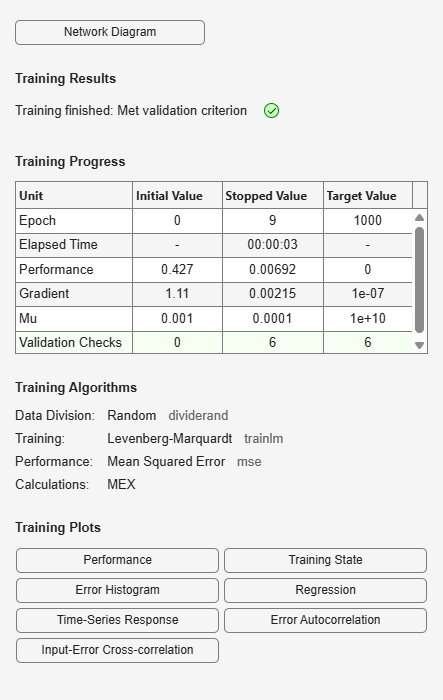# plotresponse

Plot dynamic network time series response

## Syntax

```plotresponse(t,y) plotresponse(t1,'name',t2,'name2',...,y) plotresponse(...,'outputIndex',outputIndex) ```

## Description

`plotresponse(t,y)` takes a target time series `t` and an output time series `y`, and plots them on the same axis showing the errors between them.

`plotresponse(t1,'name',t2,'name2',...,y)` takes multiple target/name pairs, typically defining training, validation and testing targets, and the output. It plots the responses with colors indicating the different target sets.

`plotresponse(...,'outputIndex',outputIndex)` optionally defines which error element is being correlated and plotted. The default is 1.

## Examples

### Plot Target and Output Time Series Data

This example shows how to use a NARX network to solve a time series problem.

```[X,T] = simplenarx_dataset; net = narxnet(1:2,20); [Xs,Xi,Ai,Ts] = preparets(net,X,{},T); net = train(net,Xs,Ts,Xi,Ai); Y = net(Xs,Xi,Ai); plotresponse(Ts,Y)```